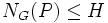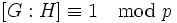# Congruence condition on index of subgroup containing Sylow-normalizer

## Statement

Suppose$G$ is a finite group,$p$ is a prime number,$P$ is a$p$-Sylow subgroup, and$H$ is a subgroup of$G$ such that$N_G(P) \le H$. In other words,$H$ contains a$p$-Sylow normalizer (?). Then, the index of$H$ in$G$ is congruent to$1$ modulo$p$.

## Facts used

1. Congruence condition on Sylow numbers
2. Sylow satisfies intermediate subgroup condition: Any$p$-Sylow subgroup of a group is also a$p$-Sylow subgroup in any intermediate subgroup.
3. Index is multiplicative

## Proof

This proof uses a tabular format for presentation. Provide feedback on tabular proof formats in a survey (opens in new window/tab) | Learn more about tabular proof formats|View all pages on facts with proofs in tabular format

Given: A finite group$G$, a prime$p$, a$p$-Sylow subgroup$P$, a subgroup$H$ of$G$ containing$N_G(P)$.

To prove:$[G:H] \equiv 1 \mod p$.

Proof:

Step no. Assertion/construction Facts used Given data used Previous steps used Explanation
1$[G:N_G(P)] \equiv 1 \pmod p$ Fact (1)$P$ is$p$-Sylow in$G$ direct
2$[H:N_G(P)] \equiv 1 \pmod p$ Facts (1), (2)$P$ is$p$-Sylow in$G$,$N_G(P) \le H \le G$$P \le N_G(P) \le H \le G$ as given. Thus, by Fact (2),$P$ is a$p$-Sylow subgroup of$H$. Also, since$N_G(P) \le H$, we have$N_G(P) = N_H(P)$. Thus, applying fact (1) to the group$H$, we get$[H:N_G(P)] = [H:N_H(P)] \equiv 1 \mod p$.
3$[G:N_G(P)] = [G:H][H:N_G(P)]$ Fact (3)$N_G(P) \le H \le G$ Fact-direct
4$[G:H] \equiv 1 \pmod p$ Steps (1), (2), (3) Go mod$p$ in Step (3) and plug in Steps (1) and (2) into the right side to obtain the conclusion.